The number pi is perhaps the most famous irrational number in the world. Most children learn about it in school, and it is popular enough to have its own day. In mathematical formulas, it is written as π, which is the Greek letter "pi." Despite this connection to Ancient Greece, people were aware of the concept of pi in earlier civilizations. Pi has also found a place in popular culture and the hearts of many, but what is Pi really and how was it discovered?

## What is Pi?

Pi is the ratio of a circle's circumference to its diameter. This is true for every circle, no matter the size. Pi is a mathematical constant and an irrational number, meaning it can't be expressed as a simple fraction. This means that the number is an infinite decimal and mathematicians have proved that the number has no repeating patterns. When working with the number the first five decimal places are generally used, which are 3.14159. Some people also shorten it further to 3.14.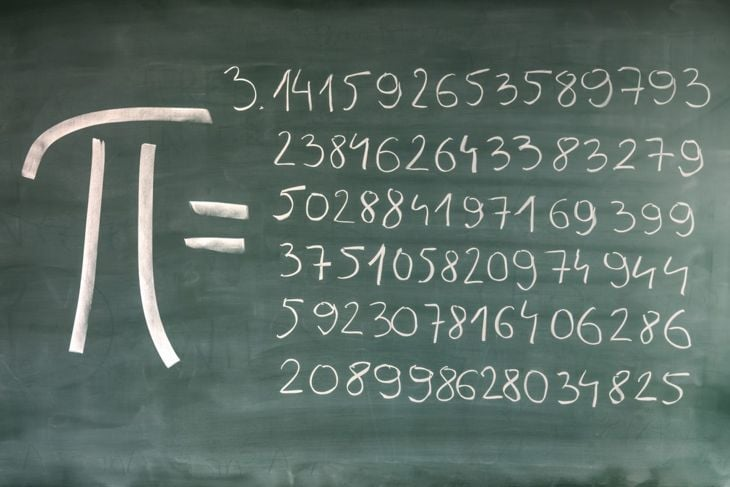domin_domin / Getty Images

## The Uses of Pi

Originally, the number of pi was mainly used in geometry. It is used in calculations to determine the circumference and area of a circle and the volume and surface area of a sphere. It is also widely used in trigonometry. In physics, pi is often used in formulas as many objects in the physical world are spherical, including planets. Number theory and statistics use pi and calculating pi is often used in computer testing.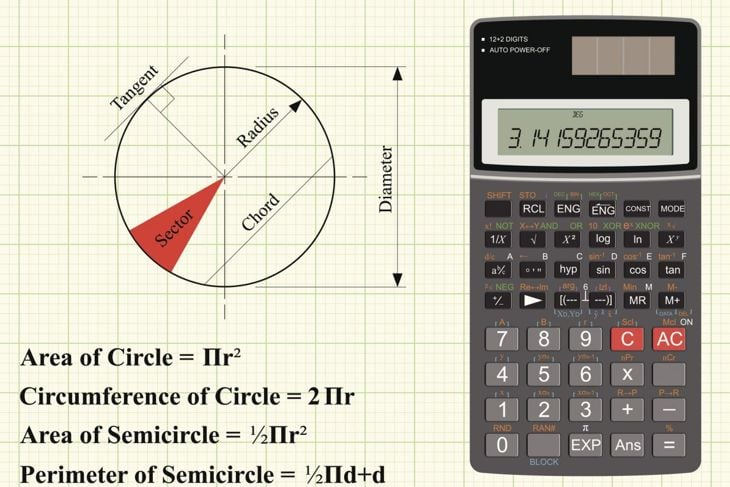Baz777 / Getty Images

## Pi in Ancient Times

Evidence shows that the ancient Egyptians and Babylonians both approximated pi. Some Egyptologists have suggested that pi was used for calculations in building the Great Pyramid of Giza, but evidence of pi doesn't appear until later. Babylonian tablets dating to 1900-1680 B.C. record an approximate number of 3.125. In Egypt, the Rhind Papyrus of around 1650 B.C. shows Egyptian mathematical calculations for the area of a circle, using the number 3.1605 for pi.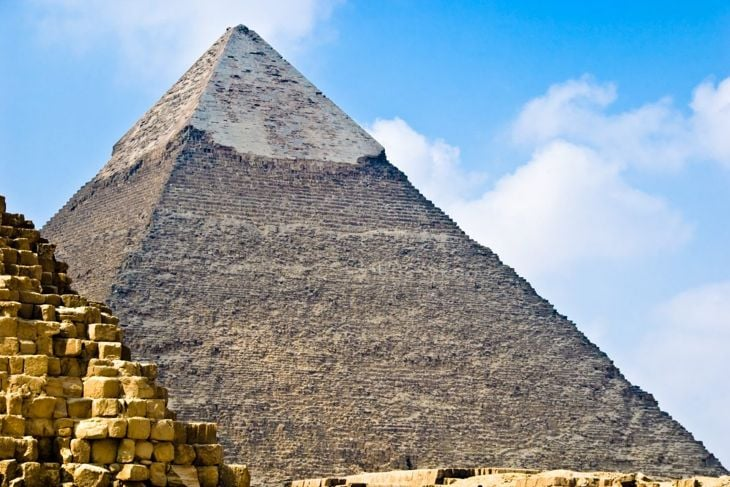timurilenk / Getty Images

## Approximations of Pi

Archimedes (287-212 B.C.) drew polygons inside of a circle and used the Pythagorean Theorem to calculate the area of the polygons. This allowed him to estimate the area of a circle. He used this to approximate the value of pi at around 3 1/7. This work is why pi is sometimes referred to as Archimedes Constant. Chinese mathematician Zu Chongzhi (429-501) used a similar process and calculated that pi equaled 355/113 and Indian mathematicians of the same era also had an approximation of pi.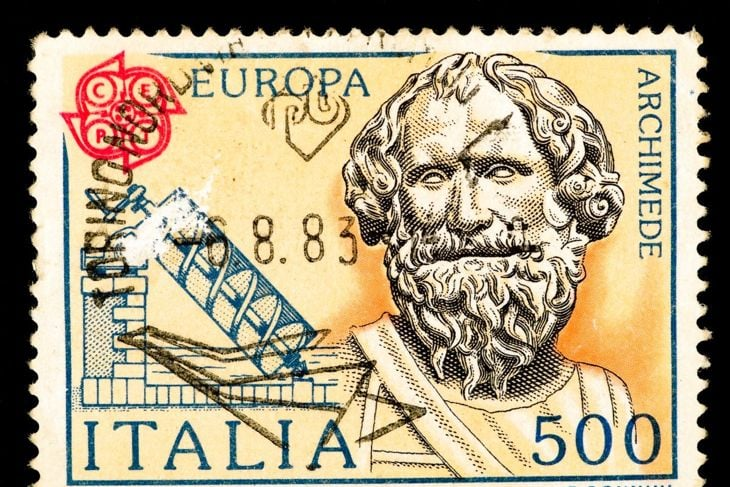blinow61 / Getty Images

## The First Exact Numbers for Pi

The Indian mathematician Mādhava was the founder of the Kerala School of Astronomy and Mathematics in the late 14th Century. He was one of the first to work with the idea of infinity. His infinite series works by adding and subtracting odd number fractions such as 1/3 and 1/5 and allowed Mādhava to calculate pi accurately to the first 13 decimal places. European mathematician Gottfried Leibniz came to the same conclusions two centuries later, and this series is now known as the Madhava–Leibniz series.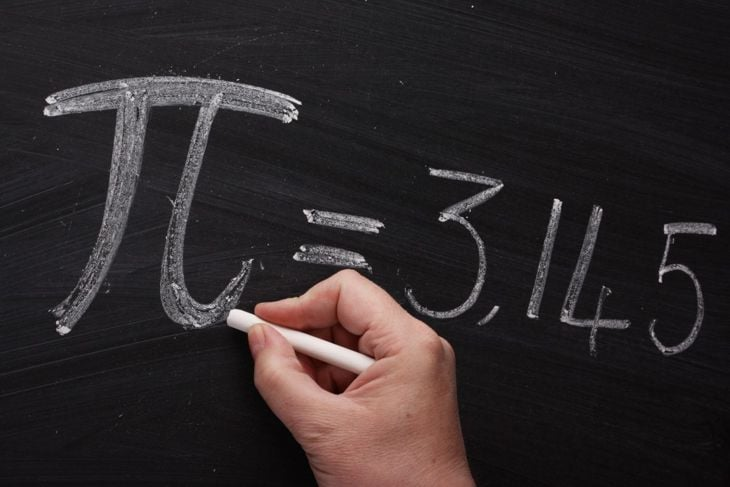Thinglass / Getty Images

## Pi in the Modern Era

During the 1700s mathematicians worked on increasing the accuracy of pi, and during this time they also proved that it is an irrational number. It was at this time that the symbol π was first used. Before this, the number was referred to by the Greek word for the periphery. In 1706, Welsh mathematician William Jones shortened this to just π, which is the first letter of the word. This became popular and was eventually adopted in all mathematical works.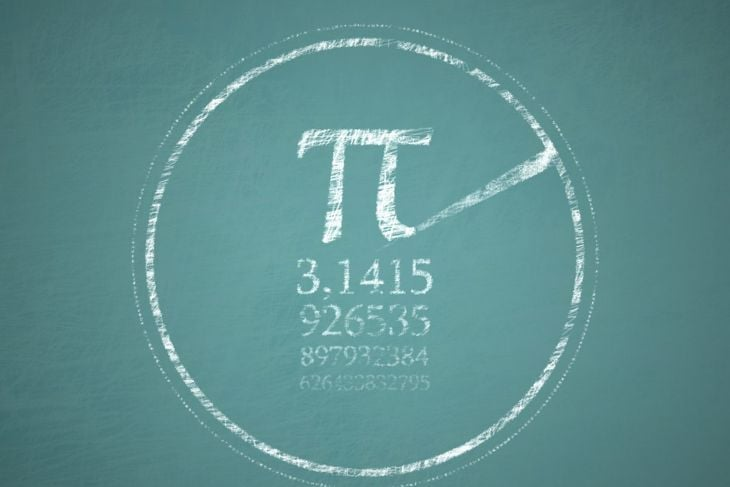alengo / Getty Images

## Pi in the Computer Age

Although a shortened number is used for most applications, people are still striving to find more decimals of pi. Prior to the advent of computers, pi was calculated to 707 decimal places. In 1961, the IBM 7090 took 8 hours and 43 minutes to calculate pi to 100,000 decimal places. The boundaries have continued to be pushed and in 2002 pi was calculated to 24 trillion decimal places. It took a University of Tokyo team 5 years to write the program that performed the calculation.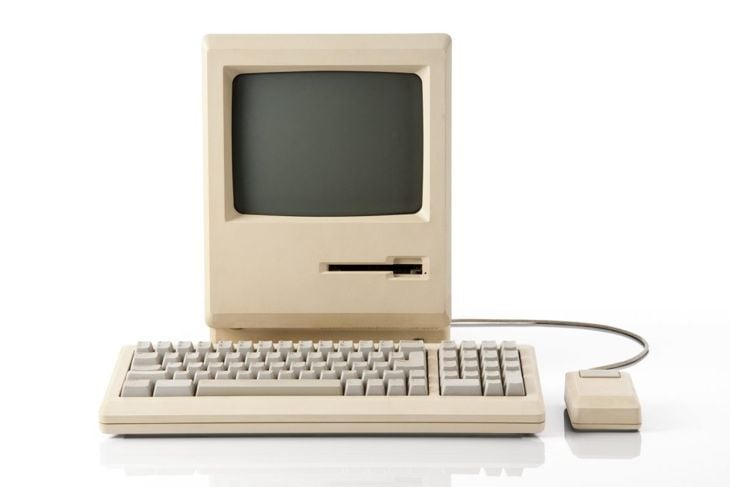Spiderstock / Getty Images

## Hand-Calculating Pi

To calculate pi by hand, start by measuring the circumference of a circular object. Wrapping string around the object gives the most accurate results. Next, measure across the circle, going through the center. This is the diameter of the circle. Divide the length of the long string by the length of the diameter to get pi. The accuracy of pi will depend on the accuracy of measurements.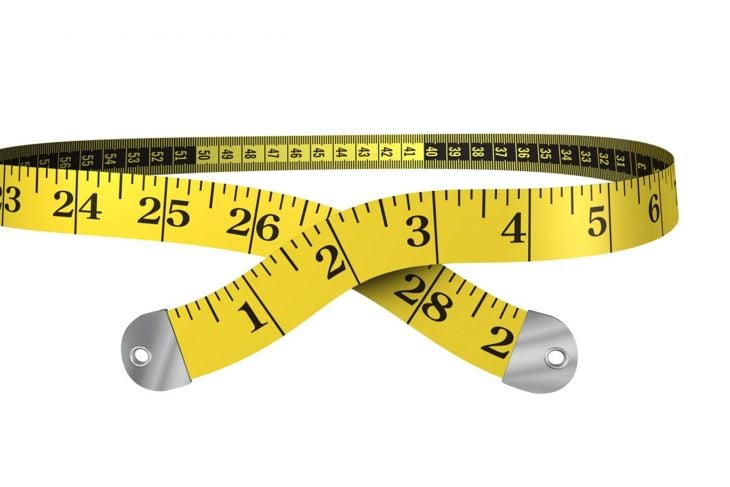MarsBars / Getty Images

## The Art of Memorizing Pi

Many people enjoy the challenge of memorizing pi. Piphilology is the art of using mnemonics to help the memorization. Pi poems, or piems, are constructed so that the number of letters in each word corresponds with the numbers of pi. The most famous poem is for 15 digits and reads: How I want a drink, alcoholic of course, after the heavy chapters involving quantum mechanics!The book Not A Wake by Michael Keith used this technique for the first 10,000 digits of pi.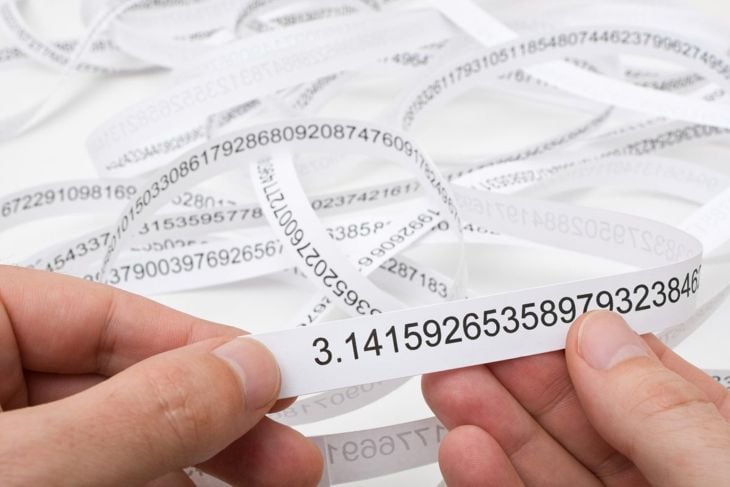shawn_hempel / Getty Images

## Pi Day

Pi Day was first celebrated in the San Francisco Exploratorium in 1988. It is celebrated on March 14 as the date equals the first three digits of pi when written as 3/14. This is also the birthday of the famous physicist Albert Einstein. In 2009, the U.S. House of Representatives passed a resolution making Pi Day a national event aimed at increasing interest in maths and science. Today, many celebrate the irrational number, often with a slice of pie.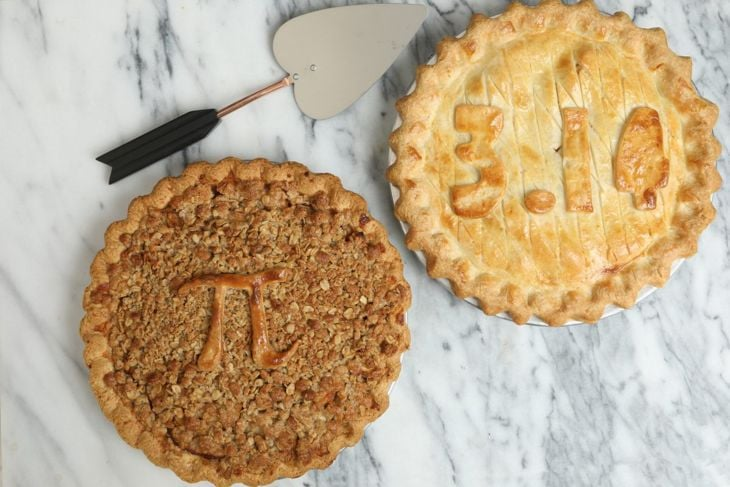DebbiSmirnoff / Getty Images

## Popular Now on Facty

### Disclaimer

This site offers information designed for educational purposes only. The information on this Website is not intended to be comprehensive, nor does it constitute advice or our recommendation in any way. We attempt to ensure that the content is current and accurate but we do not guarantee its currency and accuracy. You should carry out your own research and/or seek your own advice before acting or relying on any of the information on this Website.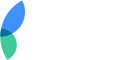Do you want to advertise on Facty.com?
Let’s talk about this! Contact us!